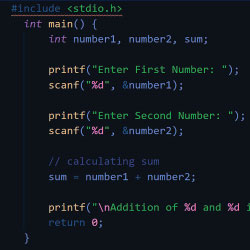# How to Generate Random Number in C#?

J

Janet

04 November 2021### Generate Random Number in C#

It is very simple to generate a Random Number in C#, There are is a Random class in C#, this class contains many methods that return various pattern random numbers, you can use those methods according to your need. In this answer, I will mention all methods of Random class with the details how that how those methods work.

In C#, in Random class, 5 methods are widely used with different kinds of parameters, Let see all methods.

1. Next()
2. Next(int)
3. Next(int, int)
4. NextDouble()
5. NextByte()

To call the above-listed methods, first, you need to create the object of the Random class and then we can call the above method with the reference of that object. Let's see how to create the object of the Random class.

Random robj=new Random()

There are three overloaded methods of Next() with different-different parameters, Let's understand each one by one.

#### 1. Next()

Generate a Random Number Between Range -2,147,483,648 to 2,147,483, 647

To generate a simple random number without any condition just use the simple Next() method. The simple Next() method returns  a random number between -2,147,483,648 to 2,147,483, 647. Let's see how to use this method.

int random_number;

Random robj = new Random();

random_number = robj.Next();

#### 2. Next(int)

Generate a Random Number, which is small than a Given Number

The method Next(int) accepts a numeric value as an argument and this method returns a positive random number smaller than the argument passed to the Next(int) method. Let's see it's working.

int random_number;

Random robj = new Random();

random_number = robj.Next(55);

The above method will return a random number between 0 to 55.

#### 3. Next(int int)

Generate a Random Number Between 2 Given Numbers

The method Next(int) is used to generate a positive random number between two numbers. This method accepts two positive numbers as arguments and then returns a random number between those arguments. Let's see how this method works.

int random_number;

Random robj = new Random();

random_number = robj.Next(120, 450);

The above method will return a random number between 120 and 450.

#### 4. NextDouble()

Generate a Floating Point Random Number

The method NextDouble() is used to generate a floating-point random number between 0.0 to 1.0, Let's see an example of this method.

int random_number;

Random robj = new Random();

random_number = robj.NextDouble()

The above method will return a random number greater than or equal to 0.0 and less than 1.0.

#### 5. NextByte()

This method is used to fill the specified array with random bytes, Let's see an example

byte[] r_Bytes = new byte;

Random robj = new Random();

rnd.NextBytes(r_Bytes );

This was all about generating a random number in C#, If you want to know how to generate a random alphanumeric string you can follow the below link.Top 10 most handsome Chinese actors
20 November 2021 | 12:00 AM